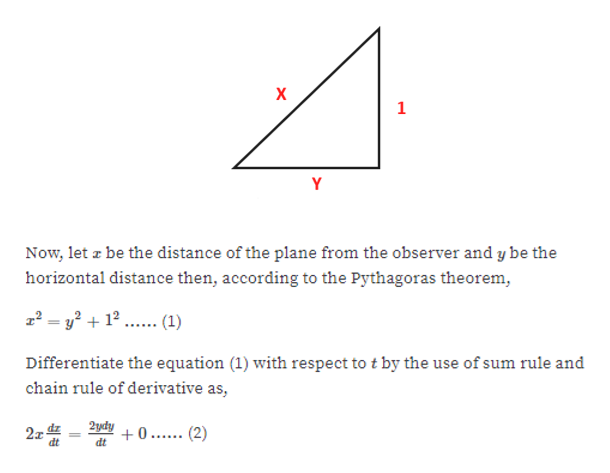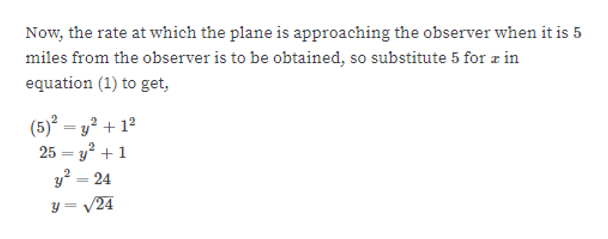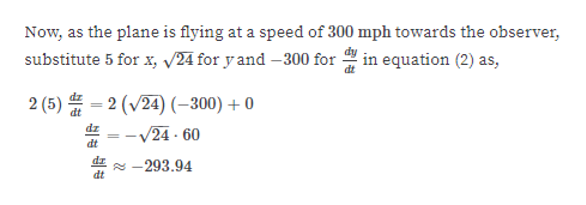# A plane is flying at a constant altitude of 1 mile and a speed of 300 mph toward an observer on the ground. How fast is the plane approaching the observer when it is 5 miles from the observer? (Round your answer to two decimal places.

Question
3 views

A plane is flying at a constant altitude of 1 mile and a speed of 300 mph toward an observer on the ground. How fast is the plane approaching the observer when it is 5 miles from the observer? (Round your answer to two decimal places.

check_circle

Step 1

The problem can be constructed as below;help_outlineImage TranscriptioncloseX 1 Y Now, let z be the distance of the plane from the observer and y be the horizontal distance then, according to the Pythagoras theorem 2y2 (1) Differentiate the equation (1) with respect to t by the use of sum rule and chain rule of derivative as, 2ydy 2 dz 0. (2) dt dt fullscreen
Step 2

For the given value of x, compute y.help_outlineImage TranscriptioncloseNow, the rate at which the plane is approaching the observer when it is 5 miles from the observer is to be obtained, so substitute 5 for 2 in equation (1) to get, (5)-22 25 y224 y=V24 fullscreen
Step 3

Using equation 2, calculate...help_outlineImage TranscriptioncloseNow, as the plane is flying at a speed of 300 mph towards the observer, substitute 5 for x, v24 for y and -300 forin equation (2) as, - 2 (V24) -300)0 2 (5) -V24 - 60 dt -293.94 dt fullscreen

### Want to see the full answer?

See Solution

#### Want to see this answer and more?

Solutions are written by subject experts who are available 24/7. Questions are typically answered within 1 hour.*

See Solution
*Response times may vary by subject and question.
Tagged in

### Derivative